Homework Help Question & Answers

# Complete the atomic orbital diagram for the ground-state electronic configuration of chlorine. Answer Bank Energy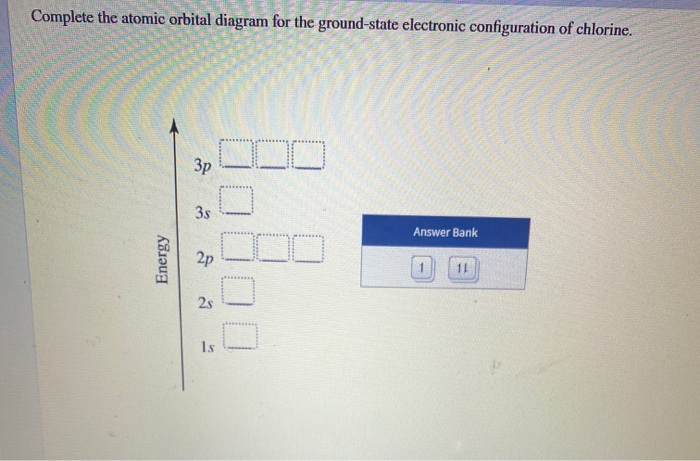Complete the atomic orbital diagram for the ground-state electronic configuration of chlorine. Answer Bank Energy

#### Homework Answers

Answer #1 ✔ Recommended Answer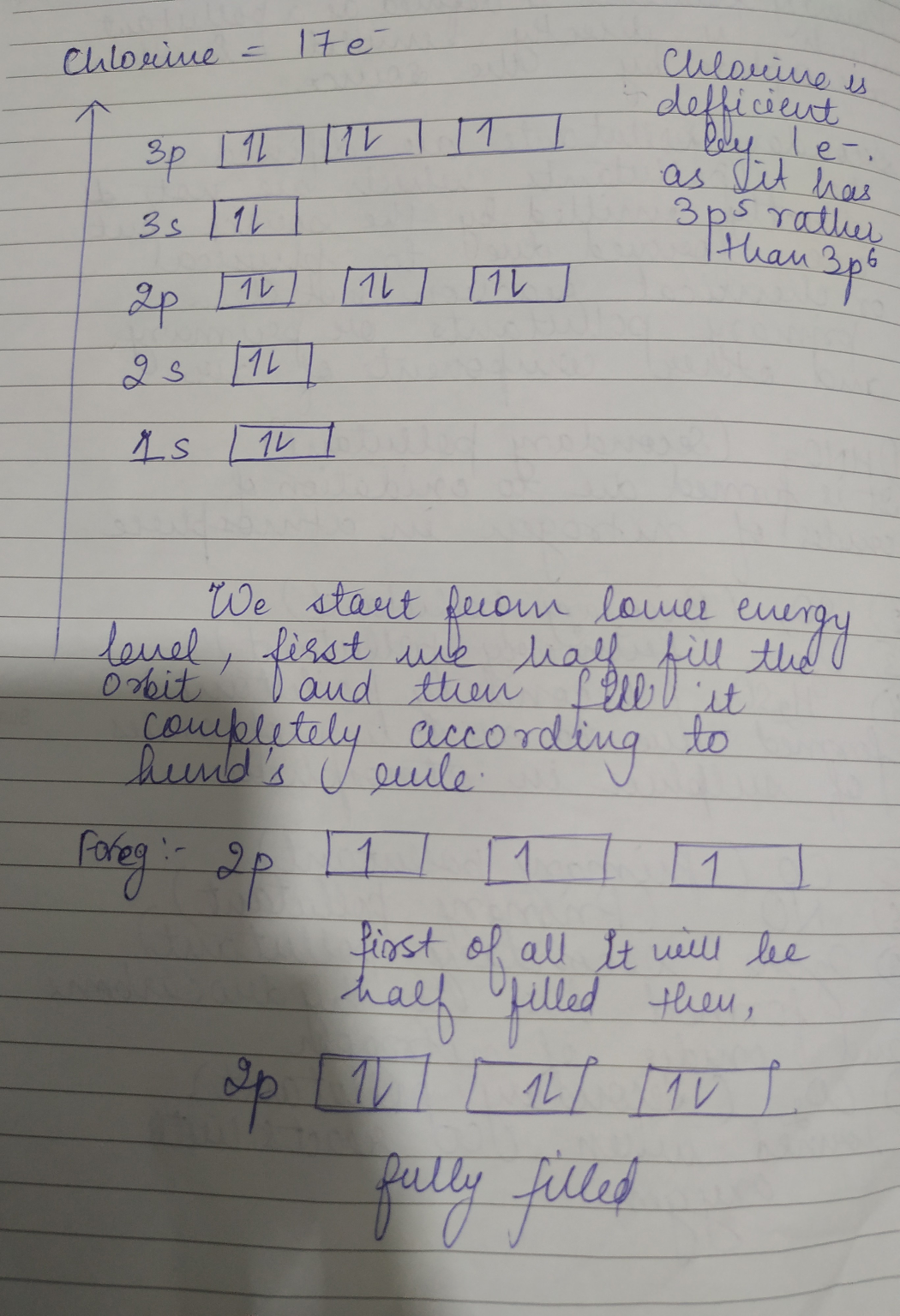Add a comment
Know the answer?
Your Answer:

#### Post as a guest

Your Name:

What's your source?

#### Earn Coin

Coins can be redeemed for fabulous gifts.

Similar Homework Help Questions
• ### 17. Write ground state electron configuration AND Orbital Box Diagram for silicon. State the number of...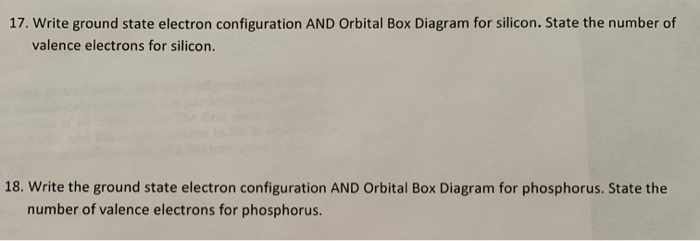17. Write ground state electron configuration AND Orbital Box Diagram for silicon. State the number of valence electrons for silicon. 18. Write the ground state electron configuration AND Orbital Box Diagram for phosphorus. State the number of valence electrons for phosphorus.

• ### 6.(18ps) (a) 6pts. What is the ground state electron configuration and orbital diagram of Nb(41)? Is...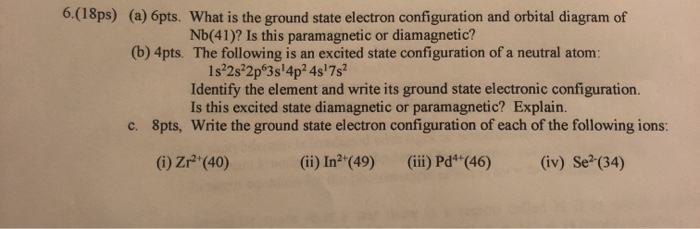6.(18ps) (a) 6pts. What is the ground state electron configuration and orbital diagram of Nb(41)? Is this paramagnetic or diamagnetic? (b) 4pts. The following is an excited state configuration of a neutral atom: 1s2s22p 3s!4p2 4s 7s Identify the element and write its ground state electronic configuration. Is this excited state diamagnetic or paramagnetic? Explain. 8pts, Write the ground state electron configuration of each of the following ions: c. (iv) Se (34) (ii) In2'(49) (ii) Pd*(46) (i) Zr (40)

• ### 6.(18ps) (a) 6pts. What is the ground state electron configuration and orbital diagram of Nb(41)? Is...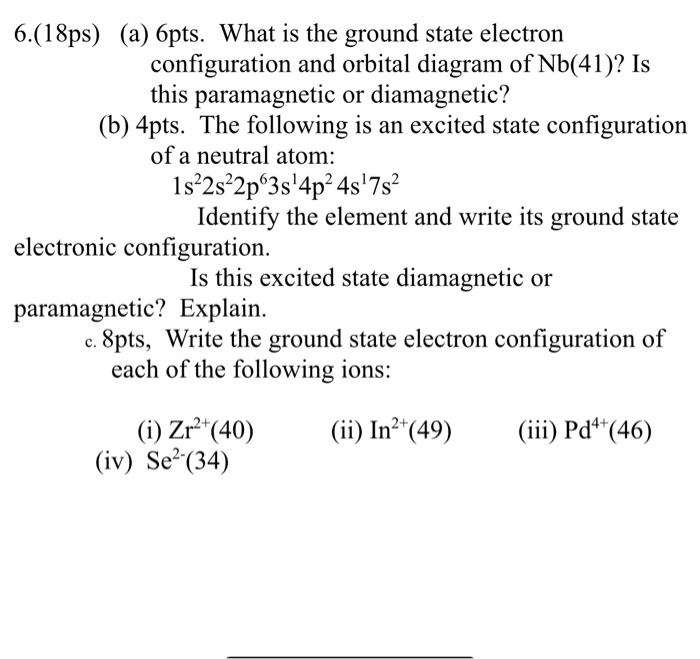6.(18ps) (a) 6pts. What is the ground state electron configuration and orbital diagram of Nb(41)? Is this paramagnetic or diamagnetic? (b) 4pts. The following is an excited state configuration of a neutral atom: 1s²2s²2pº3s'4p2 45'7s Identify the element and write its ground state electronic configuration. Is this excited state diamagnetic or paramagnetic? Explain. c. 8pts, Write the ground state electron configuration of each of the following ions: (ii) In2+(49) (iii) Pd4+(46) (i) Zr2+(40) (iv) Se?(34)

• ### 6.(18ps) (a) 6pts. What is the ground state electron configuration and orbital diagram of Nb(41)? Is...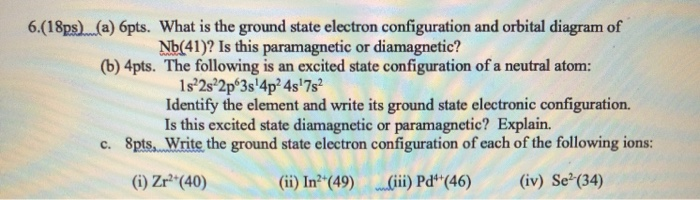6.(18ps) (a) 6pts. What is the ground state electron configuration and orbital diagram of Nb(41)? Is this paramagnetic or diamagnetic? (b) 4pts. The following is an excited state configuration of a neutral atom: 1s 2s 2p 3s'4p24s'7s2 Identify the element and write its ground state electronic configuration. Is this excited state diamagnetic or paramagnetic? Explain. c. Spts. Write the ground state electron configuration of each of the following ions: (1) Zr?*(40) (ii) In?*(49) Ciii) Pd**(46) (iv) Se?(34)

• ### Considering the generic atomic orbital energy diagram; rank the three proposed electronic transitions-1s → 2s ,...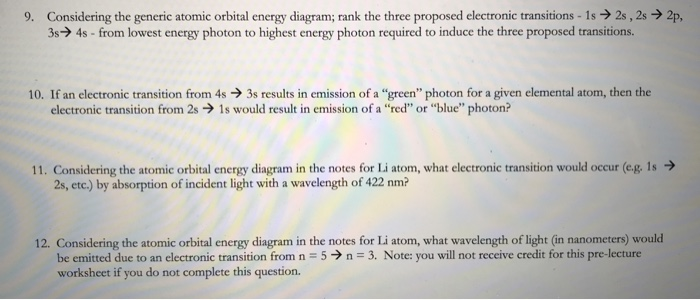Considering the generic atomic orbital energy diagram; rank the three proposed electronic transitions-1s → 2s , 2s → 2p 34s-from lowest energy photon to highest energy photon required to induce the three proposed transitions. 9. 10. If an electronic transition from 4s3s results in emission of a "green" photon for a given elemental atom, then the electronic transition from 2s 1s would result in emission of a "red" or "blue" photon? 11. Considering the atomic orbital energy diagram in the...

• ### Draw the molecular orbital of Kr2 (+2) and determine the ground state electronic configuration, bond order,...

Draw the molecular orbital of Kr2 (+2) and determine the ground state electronic configuration, bond order, magnetic properties, HOMO and LUMO of the molecule.

• ### 1)Complete the atomic orbital (AO) and molecular orbital (MO) energy diagram for Li2+ and Li2-

1)Complete the atomic orbital (AO) and molecular orbital (MO) energy diagram for Li2+ and Li2-

• ### 3. Part 2. Show your calculation or explanation for (b) and (c). (a) (3pt) The Molecular Orbital energy diagram of NO (nitrogen monoxide) is given in the right figure. Write the electron configur...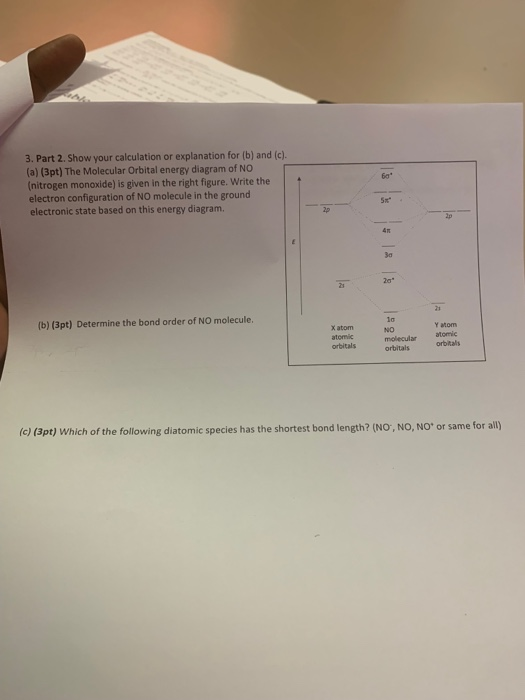3. Part 2. Show your calculation or explanation for (b) and (c). (a) (3pt) The Molecular Orbital energy diagram of NO (nitrogen monoxide) is given in the right figure. Write the electron configuration of NO molecule in the ground electronic state based on this energy diagram. 6o" Sx 20" 21 Y atom orbitals lo NO molecular atomic (b) (3pt) Determine the bond order of NO molecule. X atom atomic orbitals orbitals (c) (3pt) Which of the following diatomic species has...

• ### (ii) Draw an energy level diagram for an electronic transition from an electronic ground state to...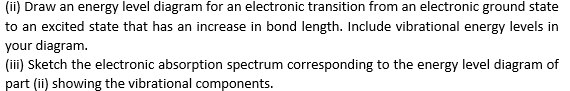(ii) Draw an energy level diagram for an electronic transition from an electronic ground state to an excited state that has an increase in bond length. Include vibrational energy levels in your diagram. (iii) Sketch the electronic absorption spectrum corresponding to the energy level diagram of part (ii) showing the vibrational components.

• ### Draw an orbital diagram showing valence electrons and write the condensed ground-state electron configuration for Se3+

Draw an orbital diagram showing valence electrons and write the condensed ground-state electron configuration for Se3+

Free Homework App

Scan Your Homework
to Get Instant Free Answers
Need Online Homework Help?

Get Answers For Free
Most questions answered within 3 hours.